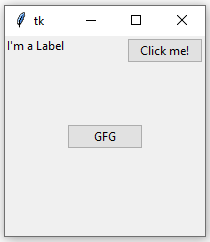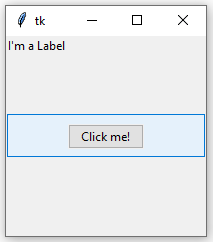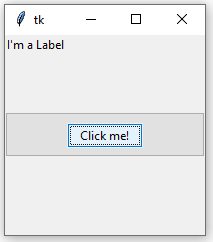Open in App
Not now

# Python | place() method in Tkinter

• Difficulty Level : Medium
• Last Updated : 22 Dec, 2022

The Place geometry manager is the simplest of the three general geometry managers provided in Tkinter. It allows you explicitly set the position and size of a window, either in absolute terms, or relative to another window. You can access the place manager through the place() method which is available for all standard widgets. It is usually not a good idea to use place() for ordinary window and dialog layouts; its simply too much work to get things working as they should. Use the pack() or grid() managers for such purposes. Syntax:

`widget.place(relx = 0.5, rely = 0.5, anchor = CENTER)`

Note : place() method can be used with grid() method as well as with pack() method. Code #1:

## Python3

 `# Importing tkinter module` `from` `tkinter ``import` `*` `from` `tkinter.ttk ``import` `*`   `# creating Tk window` `master ``=` `Tk()`   `# setting geometry of tk window` `master.geometry("``200x200``")`   `# button widget` `b1 ``=` `Button(master, text ``=` `"Click me !")` `b1.place(relx ``=` `1``, x ``=``-``2``, y ``=` `2``, anchor ``=` `NE)`   `# label widget` `l ``=` `Label(master, text ``=` `"I'm a Label")` `l.place(anchor ``=` `NW)`   `# button widget` `b2 ``=` `Button(master, text ``=` `"GFG")` `b2.place(relx ``=` `0.5``, rely ``=` `0.5``, anchor ``=` `CENTER)`   `# infinite loop which is required to` `# run tkinter program infinitely` `# until an interrupt occurs` `mainloop()`

Output:When we use pack() or grid() managers, then it is very easy to put two different widgets separate to each other but putting one of them inside other is a bit difficult. But this can easily be achieved by place() method. In place() method, we can use in_ option to put one widget inside other. Code #2:

## Python3

 `# Importing tkinter module` `from` `tkinter ``import` `*` `from` `tkinter.ttk ``import` `*`   `# creating Tk window` `master ``=` `Tk()`   `# setting geometry of tk window` `master.geometry("``200x200``")`   `# button widget` `b2 ``=` `Button(master, text ``=` `"GFG")` `b2.pack(fill ``=` `X, expand ``=` `True``, ipady ``=` `10``)`   `# button widget` `b1 ``=` `Button(master, text ``=` `"Click me !")`   `# This is where b1 is placed inside b2 with in_ option` `b1.place(in_``=` `b2, relx ``=` `0.5``, rely ``=` `0.5``, anchor ``=` `CENTER)`   `# label widget` `l ``=` `Label(master, text ``=` `"I'm a Label")` `l.place(anchor ``=` `NW)`   `# infinite loop which is required to` `# run tkinter program infinitely` `# until an interrupt occurs` `mainloop()`

Output: In below images notice that one button is placed inside the other.My Personal Notes arrow_drop_up
Related Articles